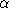## CGAL::Delaunay_mesh_size_criteria_2<CDT>

### Definition

The class Delaunay_mesh_size_criteria_2<CDT> is a model for the MeshingCriteria_2 concept. The shape criterion on triangles is given by a bound B such that for good triangles (r)/(l)B where l is the shortest edge length and r is the circumradius of the triangle. By default, B=sqrt(2), which is the best bound one can use with the guarantee that the refinement algorithm will terminate. The upper bound B is related to a lower boundmin on the minimum angle in the triangle:

 sinmin = (1)/(4 B2)

so B=sqrt(2) corresponds tomin20.7 degrees.

This traits class defines also a size criteria: all segments of all triangles must be shorter than a bound S.

#include <CGAL/Delaunay_mesh_size_criteria_2.h>

### Creation

Delaunay_mesh_size_criteria_2<CDT> traits;
Default constructor. B=sqrt(2). No bound on size

 Delaunay_mesh_size_criteria_2 traits ( double b = 0.125, double S = 0);
Construct a traits class with bound B=sqrt((1)/(4 b)). If S0, the size bound is S. If S = 0, there is no bound on size.